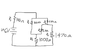# Current division in a series-parallel circuit

Ketchup4684

## Homework Statement

Determine the current through each circuit element

## Homework Equations

V = IR (Ohm's Law),

## The Attempt at a Solution

I1 is .06A after plugging 6V and 100 ohms into V = IR, but I can't figure out the rest, because I don't know how the current splits. The left path obviously has less resistance, so more current will flow through it, but I don't know how to calculate how the .06A will split because of that. Since that 50 ohm wire connects the 2 paths i don't know how it affects the total resistances of each.

#### Attachments

•series-paraller circuit.png
8.7 KB · Views: 401

Homework Helper
Hello Ketchup,It's a little more complicated than you make it out: 6V/100##\Omega## isn't right, because that would mean 6 V over 100##\Omega## and zero V over the rest of the circuit -- so zero current ##\Rightarrow## contradiction !

You know about Kirchoffs laws ? This exercise assumes you do -- or else that you discover one of them !

•scottdave